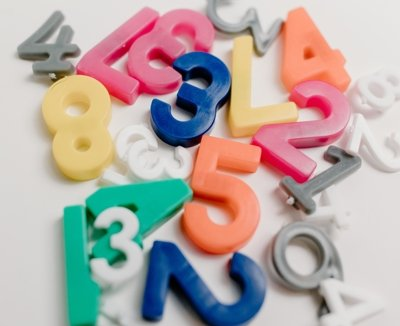Binary to hexadecimal conversion is the process of converting a binary number (base-2) to a hexadecimal number (base-16) by grouping the binary digits into sets of four and replacing each group with its corresponding hexadecimal digit.

To convert a binary number to a hexadecimal number, follow these steps:

1. Group the binary number into sets of four digits starting from the rightmost digit. Add zeros to the left if necessary to complete the last group of four digits.
2. Convert each group of four binary digits to a single hexadecimal digit according to the following table:
1. Write the hexadecimal digits in order from left to right to get the final hexadecimal equivalent of the binary number.

Find the hexadecimal value of 10101111000110000101:

Exemple in javascript code:

``````function binaryToHex(binary) {
let groups = binary.match(/.{1,4}/g);
let hexDigits = groups.map(group => {
let decimal = parseInt(group, 2);
let hex = decimal.toString(16).toUpperCase();
return hex;
});
let hexString = hexDigits.join('');
return hexString;
}
``````

Example in C# Code:

``````public string BinaryToHex(string binaryString)
{
int decimalValue = Convert.ToInt32(binaryString, 2);
string hexString = decimalValue.ToString("X");
return hexString;
}
``````

Categories:

Tags:

## Comentários

Nenhum comentário para mostrar.